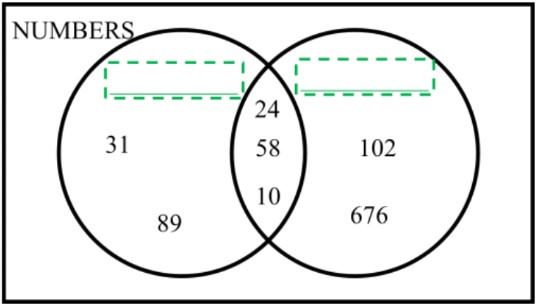# Organizing data using Venn diagrams

### Organizing data using Venn diagrams

In this lesson, we will learn:

• How to describe common characteristics/attributes in groups of objects
• What the regions of the Venn Diagram mean in order to sort objects by their attributes
• The vocabulary "AND", "OR", and "NOT" within the context of Venn Diagrams

Notes:

• Venn Diagrams organize data by sorting by characteristics ("attributes")
• Circles are used to group objects by these characteristics
• Objects inside the circle have the attribute
• Objects outside the circle do NOT have the attribute
• Objects with more than 1 attribute go into the overlapping part of attribute circles they belong to
• A large rectangle surrounds all circles and objects, it represents all the objects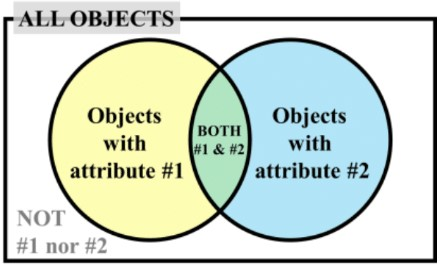• Alphabet letters are given to objects to save space when sorting in the Venn diagram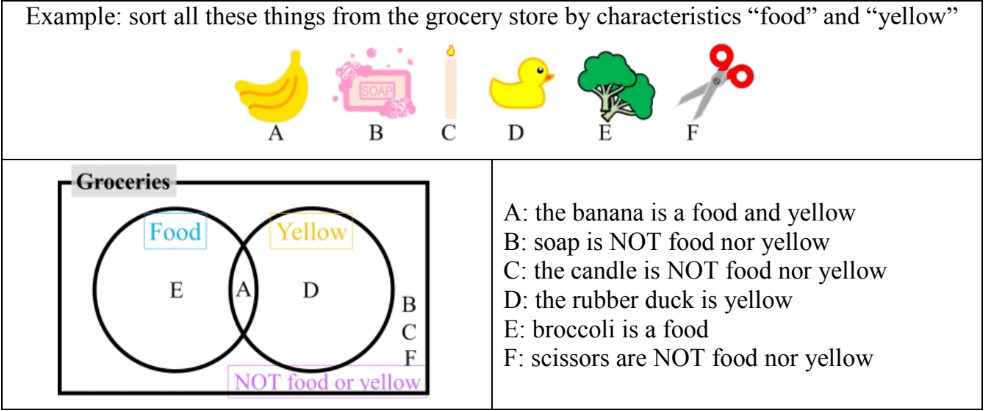• The vocabulary words "AND", "OR", and "NOT" have special meanings for sorting:
• AND = means must have both attributes
• OR = means having either attribute
• NOT = means not including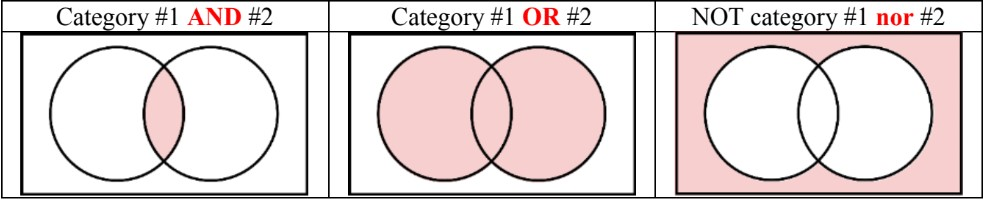#### Lessons

• Introduction
Introduction to Venn Diagrams:
a)
Understanding attributes

b)
Sorting objects with Venn diagram circles

c)
Review: regions of the Venn diagram

• 1.
Recognizing and discriminating attributes of shapes
Write the letter of the shape(s) that matches the attribute of: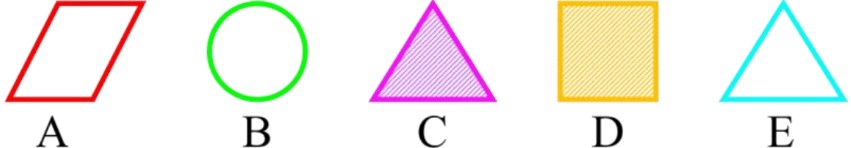a)
triangle

b)
triangle OR striped

c)
triangle AND striped

d)
not a triangle

• 2.
Organizing objects into a Venn Diagram
Sort the object into the proper category in the Venn diagram. Write each letter in the correct region
a)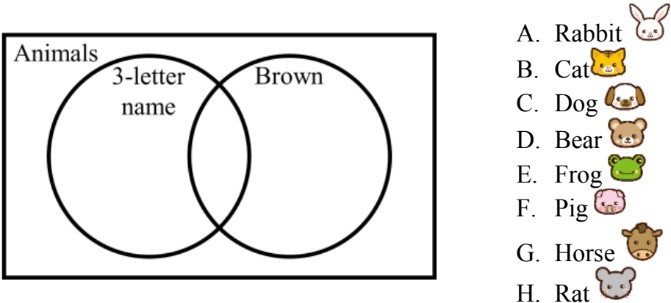b)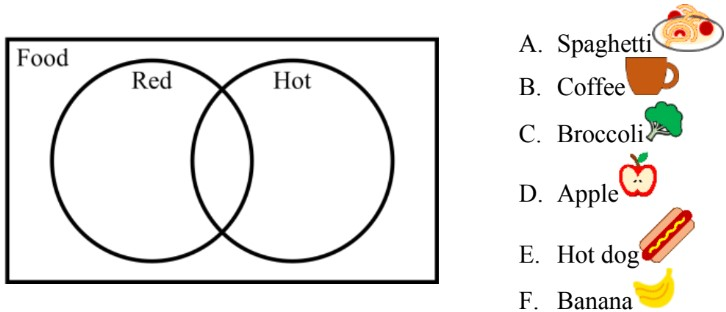c)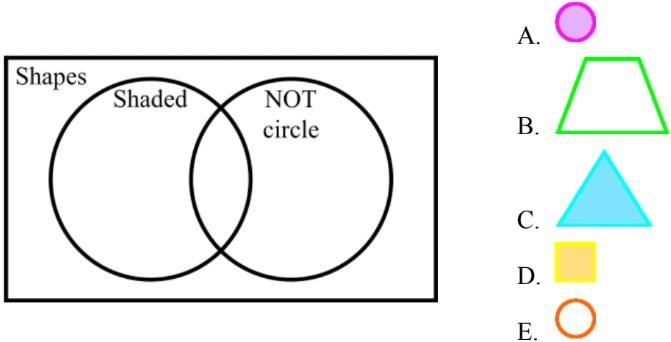• 3.
Deducing Venn diagram categories
What are the categories for the Venn diagram circles?
a)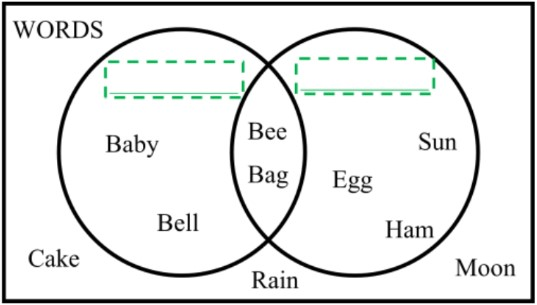b)